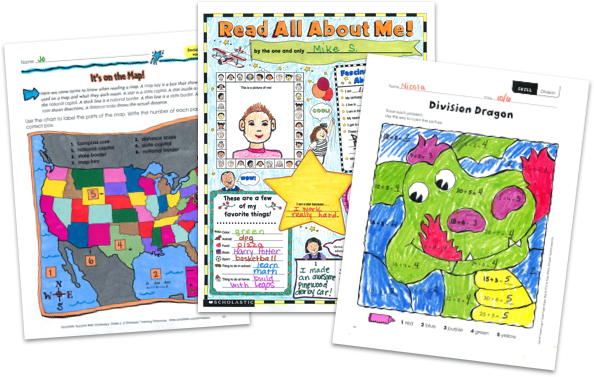MY FILE CABINET# Free Printable Math Worksheets from Scholastic TeachablesScholastic Teachables (formerly Scholastic Printables) has hundreds of printable math worksheets for grades Pre-K through 8 that you can try for FREE with a 30-day trial. Our math worksheets span a wide range of essential math skills—at every level for every learner! Sign up today and get instant online access to printable math worksheets, as well as math lesson plans, practice pages, and more to cover all grades and skills.

Scholastic Teachables (formerly Scholastic Printables) has hundreds of printable math worksheets for grades Pre-K through 8 that you can try for FREE with a 30-day trial. Our math worksheets span a wide range of essential math skills—at every level for every learner! Sign up today and get instant online access to printable math worksheets, as well as math lesson plans, practice pages, and more to cover all grades and skills.

## Our Printable Math Worksheets Cover:

• Charts and Graphs
• Data Analysis
• Decimals
• Early Math
• Estimation
• Fractions
• Geometry
• Glyphs
• Logic and Problem Solving
• Math Fluency and Intervention
• Money
• Multiplication and Division
• Numbers and Counting
• Number Sense
• Order of Operations
• Patterns
• Percents, Ratios, Proportions and Scale
• Place Value
• Probability & Statistics
• Real-World Math
• Time and Measurement
• Types of Numbers
• Word Problems

## Sample Printable Math Worksheets

Check out hundreds of Scholastic Teachables' printable math worksheets—FREE (with a 30-day trial or subscription). Sign up and you'll have access to Scholastic's more than 30,000 award-winning printables for all subjects and grades, including worksheets, lesson plans, practice pages, and more.

Here's a sample of worksheets for you to try in your class.

## Our Printable Math Worksheets Cover:

• Charts and Graphs
• Data Analysis
• Decimals
• Early Math
• Estimation
• Fractions
• Geometry
• Glyphs
• Logic and Problem Solving
• Math Fluency and Intervention
• Money
• Multiplication and Division
• Numbers and Counting
• Number Sense
• Order of Operations
• Patterns
• Percents, Ratios, Proportions and Scale
• Place Value
• Probability & Statistics
• Real-World Math
• Time and Measurement
• Types of Numbers
• Word Problems
• And More!
• Charts and Graphs
• Data Analysis
• Decimals
• Early Math
• Estimation
• Fractions
• Geometry
• Glyphs
• Logic and Problem Solving
• Math Fluency and Intervention
• Money
• Multiplication and Division
• Numbers and Counting
• Number Sense
• Order of Operations
• Patterns
• Percents, Ratios, Proportions and Scale
• Place Value
• Probability & Statistics
• Real-World Math
• Time and Measurement
• Types of Numbers
• Word Problems
• And More!

## Sample Printable Math Worksheets

Check out hundreds of Scholastic Teachables' printable math worksheets—FREE (with a 30-day trial or subscription). Sign up and you'll have access to Scholastic's more than 30,000 award-winning printables for all subjects and grades, including worksheets, lesson plans, practice pages, and more.

Here's a sample of worksheets for you to try in your class.## Additional Alphabet Worksheets (Subscription Required)

Try FREE for 30 days!

If you are not 100% satisfied cancel during the FREE trial and owe nothing. If you are not 100% satisfied cancel during the FREE trial and owe nothing.